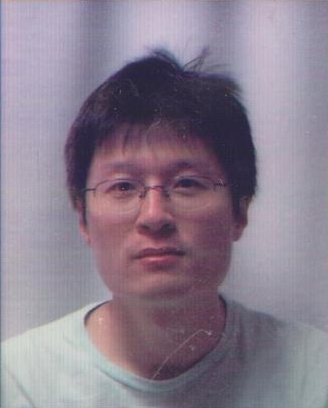Liang Kong

Institute for Quanfum Science and Engineering

I am working in the field of mathematical physics. I am interested in category theory, the mathematical theory of conformal field theories and topological field theories, and their applications in both physics and mathematics.

Research

Mathematical Physics: topological/conformal quantum field theories, topological orders, category theory.